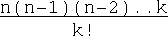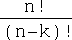Chris Umbel

# A little bit o' ErlangAlthough it's not the subject of this article I've been fooling around with CouchDB a bit lately. CouchDB is perhaps most well known for being written in Erlang, a functional language developed by Ericsson. All of the fiddling around really got me interested in actually hacking out some Erlang to evaluate any practical purpose it may have to me.

I've messed around with functional languages in the past, namely F#, Caml and quite a bit of Lisp, but this was all in my spare time with no real goal in mind. In short, I am not an experienced functional programmer. I am familiar enough that if I bend my brain I can more or less get into the functional mindset although it certainly doesn't feel natural to me. Due to this please don't consider the code I'm going to share the work of anyone knowledgeable. If anything I'm looking for feedback on how to actually do this stuff right.

Disclaimer out of the way I figured I'd post the two little programs I whipped out to learn Erlang.

Classic Mathematical Example

Considering functional languages lend themselves to mathematics by their nature and I've always enjoyed probability & statistics I figured I'd take a stab whipping out some code to compute combinations and permutations. The first step to accomplishing either of these are a basic function to compute factors.

```fact(M, 0) ->
1;

fact(M, A) ->
M * fact(M - 1, A - 1).
```

This is actually one function named fact. The first parameter, "M", will serve as the number to factor and the second, "A", will serve as an accumulator which controls how many factors I'll compute (when M = A we get a factorial). Note how the first declaration ends with a semicolon. That indicates that there's more function to come. The period at the end of the second declaration indicates that I'm done defining my function. Essentially the Erlang VM will execute the first definition recursively until the accumulator reaches zero in which case it'll execute the first definition.

Now I'll define functions to actually compute combinations and permutations

```combo(N, K) ->
fact(N, K) / fact(K, K).

permu(N, K) ->
fact(N, N) / fact(N - K, N - K).
```

which represent nCkand nPkrespectively.

Now I'll actually package it up into a module named probability (in a file named probability.erl) so I can compile and use it:

```-module(probability).
-export([combo/2]).
-export([permu/2]).

fact(M, 0) ->
1;

fact(M, A) ->
M * fact(M - 1, A - 1).

combo(N, K) ->
fact(N, K) / fact(K, K).

permu(N, K) ->
fact(N, N) / fact(N - K, N - K).
```

Now from the Erlang shell I can compile my code with

```> c(probability).
```

and test it with

```> probability:combo(8, 6).
```

resulting in

```28.0
```

and

```> probability:permu(10, 3).
```

producing

```720.0
```

A more fun example

Ok, well that's all fun and games, but... Actually, no. It's not much fun at all. So I figured I'd whip up a function to retrieve the status of a user on twitter.

```-module(twitterstatus).
-export([getstatus/1]).

%% initialize internet services
application:start(inets),

UserAgent = "Erlang Status Lookerupper",
%% format a URL
Url = "http://twitter.com/users/" ++ UserName ++ ".xml",

%% perform the HTTP request to twitter
{ok, {{HttpVer, Code, Msg}, Headers, Body}} =
http:request(get, {Url, [{"User-Agent", UserAgent}]}, [], []),

%% parse the response XML
{RootElement, _RemainingText = ""} =
xmerl_scan:string(Body),

%% pull the status text out
[{_,[A|B],_,[], StatusText, _}] =
xmerl_xpath:string("/user/status/text/text()", RootElement),

%% return the status text
StatusText.
```

Which can be called like

```> twitterstatus:getstatus("chrisumbel").
```

Conclusion

Will this be useful to me? I'm not sold either way yet. I found it to be concise but I think it'll take me a while to get used to reading it. I suppose I was productive enough, however, that I'll continue to explore it in more depth if time permits.

Sun Aug 23 2009 23:08:00 GMT+0000 (UTC)